1. (Smallest magic square, 3 x 3)
Using the numbers 1 to 9, fill in the square so that the rows across, down and diagonally all add up to 15.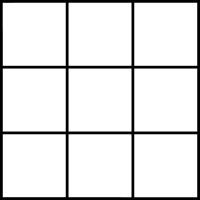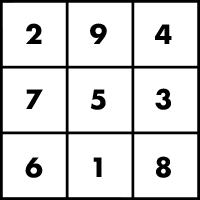2. Construct a magic square of 3 x 3 which has a magic constant of 30.
[Hint. Central box must have 30/3 = 10; use the numbers 6 to 14].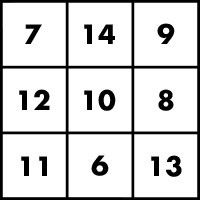3. Using only the numbers 4 through 12, fill in the circles so that the numbers on each straight line add up to 24.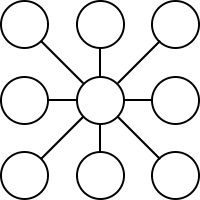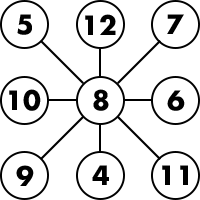4. Fill in the circles with the numbers from 2 to 6 so that each side of the triangle add up to 10.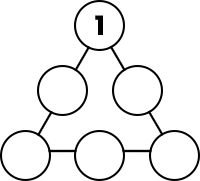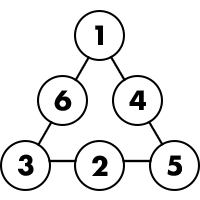5. Fill in the circles with the numbers from 1 to 8 so that each side of the square adds up to 13.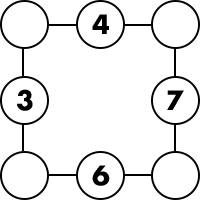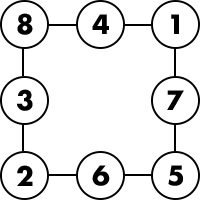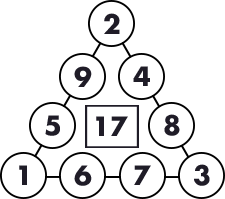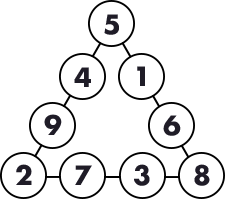Sum of each side = 20